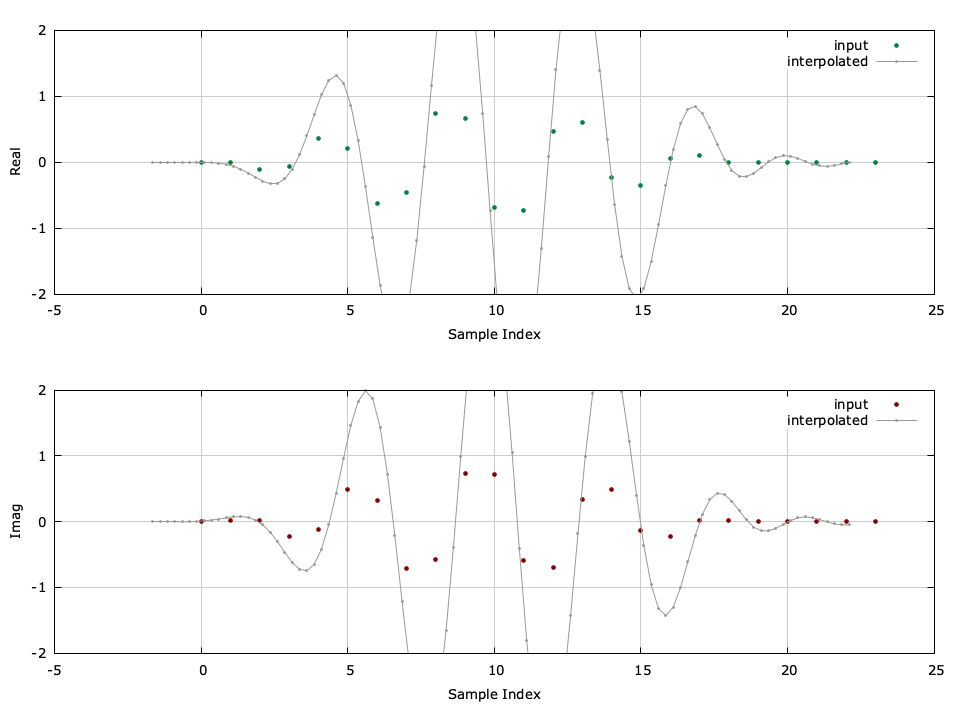# Infinite Impulse Response Interpolator (iirinterp)

The iirinterp object implements an infinite impulse response (IIR) interpolator with an integer output-to-input resampling ratio. An example of the iirinterp interface is listed below.

#include <liquid/liquid.h>

int main() {
// options
unsigned int M = 4;     // decimation factor
unsigned int n = 21;    // filter length

// design filter and create decimator object
iirdecim_crcf q = iirdecim_crcf_create_default(M,n);

// generate input signal and decimate
float complex x[M];         // input samples
float complex y;            // output sample

// run decimator (repeat as necessary)
{
iirdecim_crcf_execute(q, x, &y);
}

// destroy decimator object
iirdecim_crcf_destroy(q);
}


Listed below is the full interface to the iirinterp family of objects. While each method is listed for iirinterp_crcf , the same functionality applies to iirinterp_rrrf and iirinterp_cccf .

• iirinterp_crcf_create(M,*b,nb,*a,na) creates a iirinterp object with a interpolation factor $$M$$ using the filter coefficients defined by $$\vec{a}$$ and $$\vec{b}$$ .
• iirinterp_crcf_create_default(M,order) creates a iirinterp object from a prototype filter with a interpolation factor $$M$$ and a filter order $$order$$ . The default filter is a Butterworth design.
• iirinterp_crcf_create_prototype(M,ftype,btype,format,order,fc,f0,Ap,As) creates a iirinterp object from a prototype with a interpolation factor $$M$$ . See iirdes for details on the filter design parameters.
• iirinterp_crcf_destroy(q) destroys a iirinterp object, freeing all internally-allocated memory.
• iirinterp_crcf_print(q) prints the parameters of a iirinterp object to the standard output.
• iirinterp_crcf_reset(q) clears the internal buffer of a iirinterp object.
• iirinterp_crcf_execute(q,x,*y) computes the output interpolation of the input sample $$x$$ and stores the output in the $$M$$ -sample buffer $$\vec{y}$$ .
• iirinterp_crcf_execute_block(q,*x,n,*y) computes the output interpolation of the input sequence $$\vec{x}$$ (which is $$n$$ samples in size) and stores the resulting $$nM$$ samples in $$y$$ .
• iirinterp_crcf_groupdelay(q,fc) computes the filter group delay at frequency $$f_c$$ .Figure [fig-filter-iirinterp]. iirinterp_crcf (interpolator) example with $$M=4$$ , compensating for filter delay.

A graphical example of the interpolator can be seen in[ref:fig-filter-iirinterp] . A detailed example program is given in examples/iirinterp_crcf_example.c , located under the main liquid project directory.